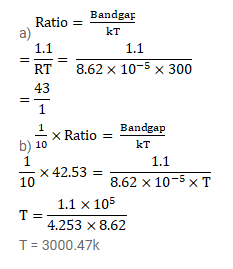# The band gap for silicon is 1.1eV. (a) Find the ratio of the band gap to kT for silicon at room temperature 300k.

Question:

The band gap for silicon is $1.1 \mathrm{eV}$.

(a) Find the ratio of the band gap to $\mathrm{kT}$ for silicon at room temperature $300 \mathrm{k}$.

(b) At what temperature does this ratio become one tenth of the value at $300 \mathrm{k}$ ? (Silicon will not retain its structure at these high temperatures.)

Solution: